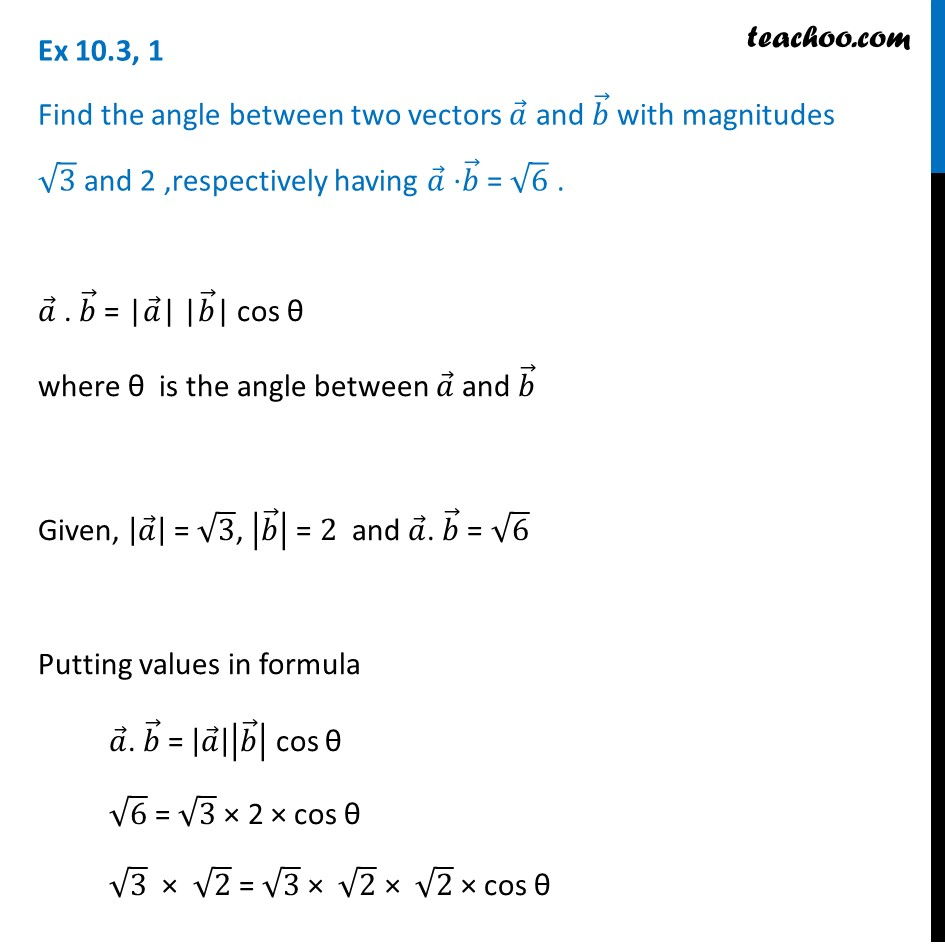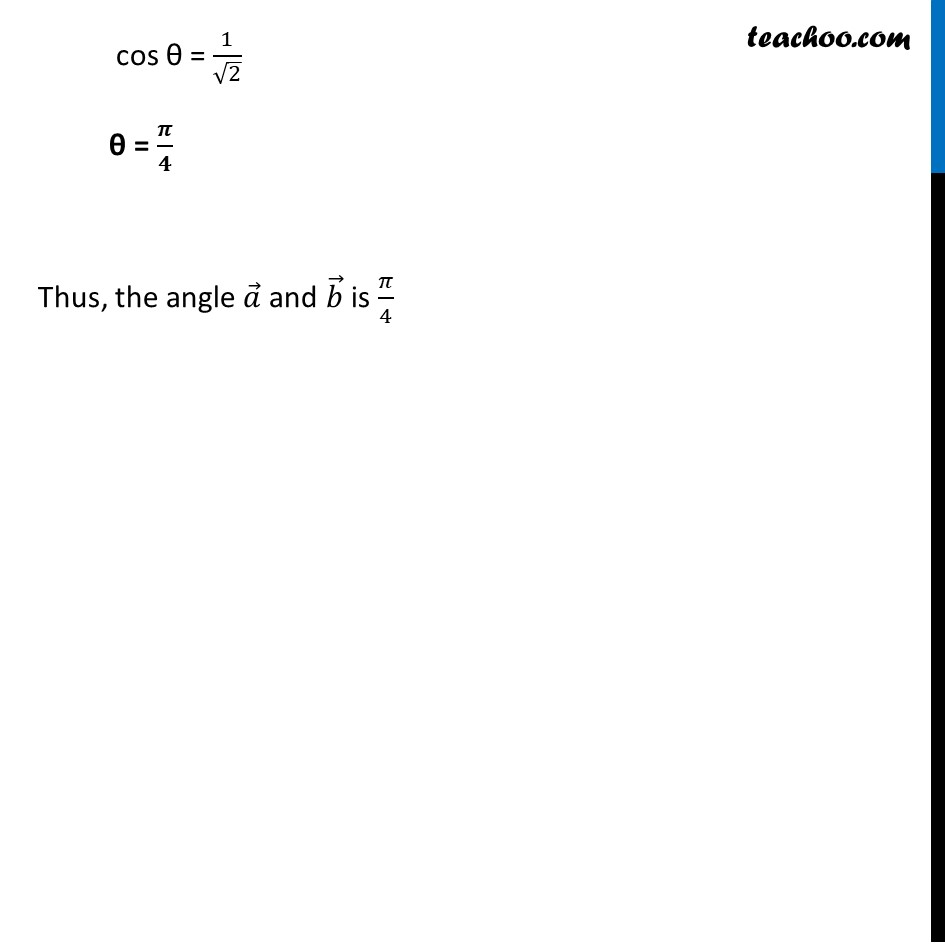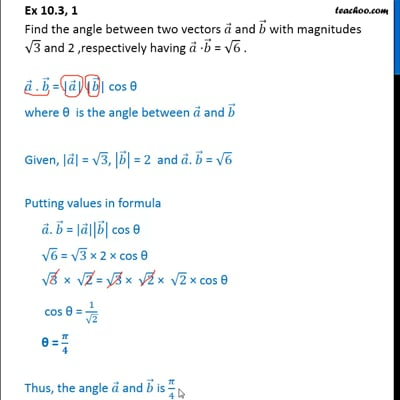Ex 10.3

Chapter 10 Class 12 Vector Algebra
Serial order wiseThis video is only available for Teachoo black users

Learn in your speed, with individual attention - Teachoo Maths 1-on-1 Class

### Transcript

Ex 10.3, 1 Find the angle between two vectors 𝑎 ⃗ and 𝑏 ⃗ with magnitudes √3 and 2 ,respectively having 𝑎 ⃗ ⋅𝑏 ⃗ = √6 . 𝑎 ⃗ . 𝑏 ⃗ = "|" 𝑎 ⃗"|" "|" 𝑏 ⃗"|" cos θ where θ is the angle between 𝑎 ⃗ and 𝑏 ⃗ Given, |𝑎 ⃗ | = √3, |𝑏 ⃗ | = 2 and 𝑎 ⃗. 𝑏 ⃗ = √6 Putting values in formula 𝑎 ⃗. 𝑏 ⃗ = |𝑎 ⃗ ||𝑏 ⃗ | cos θ √6 = √3 × 2 × cos θ √3 × √2 = √3 × √2 × √2 × cos θ cos θ = 1/√2 θ = 𝝅/𝟒 Thus, the angle 𝑎 ⃗ and 𝑏 ⃗ is 𝜋/4